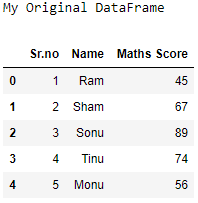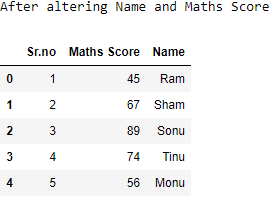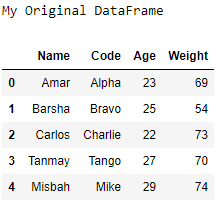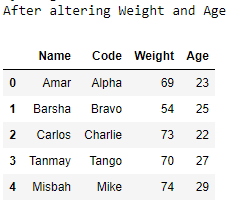Related Articles

# Change the order of a Pandas DataFrame columns in Python

• Last Updated : 11 Aug, 2021

Let us see how to change the order of a DataFrame’s columns.

Algorithm :

• Create a DataFrame.
• Reassign the same DataFrame with the order of the columns changed.
• Print the DataFrame.

Example 1 :

## Python3

 `# importing the modules``import` `pandas as pd``import` `numpy as np` `# creating the DataFrame``my_data ``=` `{``'Sr.no'``: [``1``, ``2``, ``3``, ``4``, ``5``],``           ``'Name'``: [``'Ram'``, ``'Sham'``, ``'Sonu'``,``                    ``'Tinu'``, ``'Monu'``],``           ``'Maths Score'``: [``45``, ``67``, ``89``, ``74``, ``56``]}``df ``=` `pd.DataFrame(data ``=` `my_data)` `# printing the original DataFrame``print``(``"My Original DataFrame"``)``print``(df)` `# altering the DataFrame``df ``=` `df[[``'Sr.no'``, ``'Maths Score'``, ``'Name'``]]` `# printing the altered DataFrame``print``(``'After altering Name and Maths Score'``)``print``(df)`

Output:Example 2 :

## Python3

 `# importing the modules``import` `pandas as pd`` ` `# creating the DataFrame``l1 ``=``[``"Amar"``, ``"Barsha"``, ``"Carlos"``, ``"Tanmay"``, ``"Misbah"``]``l2 ``=``[``"Alpha"``, ``"Bravo"``, ``"Charlie"``, ``"Tango"``, ``"Mike"``]``l3 ``=``[``23``, ``25``, ``22``, ``27``, ``29``]``l4 ``=``[``69``, ``54``, ``73``, ``70``, ``74``]``df ``=` `pd.DataFrame(``list``(``zip``(l1, l2, l3, l4)))``df.columns ``=``[``'Name'``, ``'Code'``, ``'Age'``, ``'Weight'``]` `# printing the original DataFrame``print``(``"My Original DataFrame"``)``print``(df)`` ` `# altering the DataFrame``df ``=` `df[[``'Name'``, ``'Code'``, ``'Weight'``, ``'Age'``]]`` ` `# printing the altered DataFrame``print``(``'After altering Weight and Age'``)``print``(df)`

Output :Attention geek! Strengthen your foundations with the Python Programming Foundation Course and learn the basics.

To begin with, your interview preparations Enhance your Data Structures concepts with the Python DS Course. And to begin with your Machine Learning Journey, join the Machine Learning – Basic Level Course

My Personal Notes arrow_drop_up